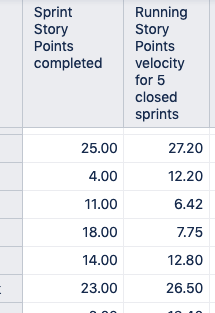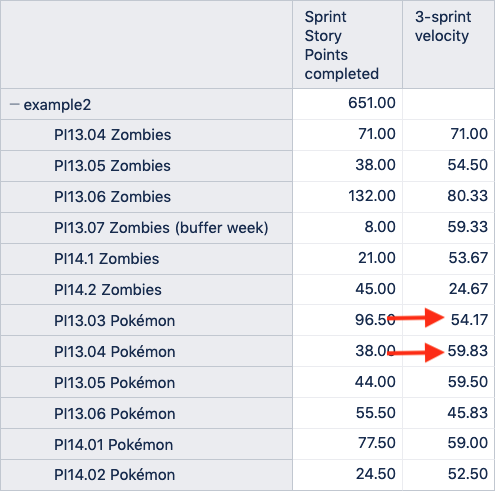# Need set of sprints from specific gorup of boards

Hi,
I am trying to create a report that totals up several metrics (e.g., story points committed, story points completed, etc) for a specific set of Sprints from within a group of related Jira Boards and I’m stuck.

Each Scrum Team has their own board. The Scrum Teams are organized into three groups within our organization. So, for example:
Group A
Team A1
Team A2
Team A3
Group B
Team B1
Team B2
Team B3

I want to be able to create reports for each Group separately and, also, combined on one report. I created a calculated member on the Sprint dimension that aggregated the boards of the individual teams, as such:
Aggregate(
{
[Sprint].[Team A1 board],
[Sprint].[Team A2 board],
[Sprint].[Team A3 board]
}
)

But I do not get the results I need. For example, when I add the measure Running Story Points velocity for 5 closed sprints, the values are incorrect. They seem to be averages of a random set of 5 sprints, not the last 5 sprints listed in the rows of the report (see below). In the example the last 5 rows add up to 70 so the running velocity should be 14 but it’s 26.5. What is wrong??I’ve seen other answers in the community for filtering based on name and other options, but none really show what I’m looking for. Any help would be greatly appreciated as I am pretty new to MDX and eazyBI

Thank you!

UPDATE:
I’ve made some progress. My intent is to create a calculated measure on the Sprint dimension and then use that dimension for my velocity calculation. I managed to do this (I don’t know if this is ideal or not so any suggestions would be welcomed). However, my velocity calculation isn’t quite correct as it uses the completed points from another team’s board to calculate the velocity for the second team.

Here’s my calculated member, called ‘example’, that works (this is based on the All Closed Sprints calculated member):

``````Aggregate({
Order(
Tail(Filter([Sprint].[Android - Zombies].Children,
[Sprint].CurrentMember.getBoolean('Closed') AND
NOT IsEmpty([Sprint].CurrentMember.get('Complete date'))),6),
[Sprint].CurrentMember.get('Start date'), BASC
),
Order(
Tail(Filter([Sprint].[Android - Pokémon].Children,
[Sprint].CurrentMember.getBoolean('Closed') AND
NOT IsEmpty([Sprint].CurrentMember.get('Complete date'))),6),
[Sprint].CurrentMember.get('Start date'), BASC
)
} )
``````

My calculated measure for velocity (average of last 3 sprints) is:

``````CASE
WHEN
[Sprint].CurrentMember is [Sprint].DefaultMember
OR
[Sprint].CurrentMember.getBoolean('Closed') AND
NOT IsEmpty([Sprint].CurrentMember.get('Complete date'))
AND [Measures].[Sprint Story Points committed] > 0
THEN
AVG(Tail(
Filter(
Cache(ChildrenSet([Sprint].[example])),
Rank([Sprint].CurrentMember, Cache(ChildrenSet([Sprint].[example]))
)),
-- only sprints with committed story points are retrieved
[Measures].[Sprint Story Points committed] > 0
), 3
),
[Measures].[Sprint Story Points completed]
)
END
``````

When I do this, the velocity calculation is accurate except where the teams ‘overlap’. In the table the two highlighted values are incorrect since they’re based on the Zombies sprints. How do I correct this?Also, any better, more efficient, elegant ideas are welcome!

Hi @jrjanis,

Try defining calculated members in the Sprint dimension for each group that aggregates the team Boards, just like you did in the original post. Next, define a new calculated measure, with the formula below:

``````CASE
WHEN
[Sprint].CurrentMember is [Sprint].DefaultMember
OR
-- for closed sprints only
[Sprint].CurrentMember.getBoolean('Closed') AND
NOT IsEmpty([Sprint].CurrentMember.get('Complete date')) AND
[Measures].[Sprint Story Points committed] > 0
THEN
AVG(
Tail(
-- filter last 5 closed sprints starting from current sprint
Filter(
Cache(
Order(
Filter(VisibleRowsSet(),
[Sprint].CurrentMember.getBoolean('Closed') AND
NOT IsEmpty([Sprint].CurrentMember.get('Complete date'))),
[Sprint].CurrentMember.get('Start date'),
BASC
)
),
Rank([Sprint].CurrentMember,
Cache(
Order(
Filter(VisibleRowsSet(),
[Sprint].CurrentMember.getBoolean('Closed') AND
NOT IsEmpty([Sprint].CurrentMember.get('Complete date'))),
[Sprint].CurrentMember.get('Start date'),
BASC
)
)
)
),
-- only sprints with committed story points are retrieved
[Measures].[Sprint Story Points committed] > 0
), 5
),
[Measures].[Sprint Story Points completed]
)
END
``````

Instead of looking at the Sprint dimension “Sprint” level members from the calculated member “All closed sprints”, it looks at all the Sprints currently on rows. The VisibleRowsSet() function is responsible for this - https://docs.eazybi.com/eazybi/analyze-and-visualize/calculated-measures-and-members/mdx-function-reference/visiblerowsset.

You can now place the Sprint dimension simultaneously on rows and pages, select the Group calculated members in pages, and display the “Sprint” level members on rows. Selecting one or more Groups will adjust the result on rows and the velocity. See a picture of a sample report below:

The eazyBI docs have more information on defining calculated members and measures - https://docs.eazybi.com/eazybijira/analyze-and-visualize/calculated-measures-and-members.

Best,
Roberts // support@eazybi.com

Thanks so much Roberts. This helps a lot!

I do have a couple of follow-on questions…
What would be the best way to limit the number of Sprints that appear in the table once the “group” is selected? Right now, ALL of the Sprints from the beginning of time appears. Let’s say I wanted to limit it to only the last 6, or maybe a set of sprints between two dates?

I know how to do this as a calculated member of the Sprint dimension but it’s not clear to me how to combine that with the group of boards member I created. Do I need to run through every permutation (e.g., Last 6 sprints for Group 1, Lasts 6 sprints for Group 2, Group 1 sprints between June and December, etc.)? That doesn’t seem like the correct/best way.

My motivation for this is that we work in Program Increments (PIs) similar to SAFe. We use fixVersion in Jira to assign PI to a Feature and Epics (not always stories). BUT, there is no association in Jira between fixVersion and sprint so I can’t simply ask eazyBI for all sprints within a given PI (fixVersion). To address this I’ve taken two steps.

First, I’ve created calculated members in Sprint called “PI-1 sprints”, “PI-2 sprints”, etc., as such

``````Aggregate({
Order(
Filter(ChildrenSet([Sprint].[All board children]),
[Sprint].CurrentMember.getBoolean('Closed') AND
NOT IsEmpty([Sprint].CurrentMember.get('End date')) AND
DateBetween([Sprint].CurrentMember.get('End date'),
'13 JAN 2020', '08 APR 2020') ),
[Sprint].CurrentMember.get('Name'),
BASC
)
})
``````

In this case [All board children] is a calculated member defined as (except for up to 46 boards depending on how I’m grouping the organization):

``````Aggregate(
{
[Sprint].[Board 1].Children,
[Sprint].[Board 2].Children,
[Sprint].[Board 3].Children
}
)
``````

This appears to work well if I’m creating a report that groups measures across all sprints or all boards. However, in some cases, I want to limit the data to a specific set of sprints (time period) for a specific set of boards. For example, out of our total of 46 boards show me only these 10 boards and the last 12 sprints grouped by PI. The result would look something like this:

To accomplish this I also created calculated measures in the Time dimension for each PI, as such, for PI-12:

``````Aggregate(
[Time].[Day].DateMembersBetween('08 JAN 2020', '07 APR 2020')
)
``````

Then I created calculated measures for each PI, like this:

``````CACHE(Aggregate(
[Time].[PI-12],
SUM(
ChildrenSet([Sprint].CurrentHierarchyMember),
[Measures].[Sprint Story Points completed]) /
SUM(
ChildrenSet([Sprint].CurrentHierarchyMember),
[Measures].[Sprint Story Points committed])
))
``````

I think I’m getting the correct, expected results, but validating this is very difficult for a number of reasons.

In any case, being as new to this as I am, I am not super confident in my approach, or my results. Sometimes I get results I can’t explain. For instance, at one point my denominator in the measure above was:

[Measures].[Sprint Story Points committed] +